# Global Average Pooling• What is pooling
• Types of pooling
• What is global average pooling
• Flatten layer vs global average pooling
• Average pooling vs global average pooling
• Advantages of global average pooling
• Comparing different pooling function with code

## What is pooling

Pooling or pooling layer is one of the building blocks of Convulational Neural Network(CNN). It is applied to the feature map which we get after applying CNN to images. Its function is to reduce the spatial size of the representation to reduce the amount of parameters and computation in the network. It is mainly used to reduce the dimensions of the feature map. Pooling also provides an approach to downsample feature maps by summarizing the features present in a region of the feature map generated by convulational layer. We can understand by this: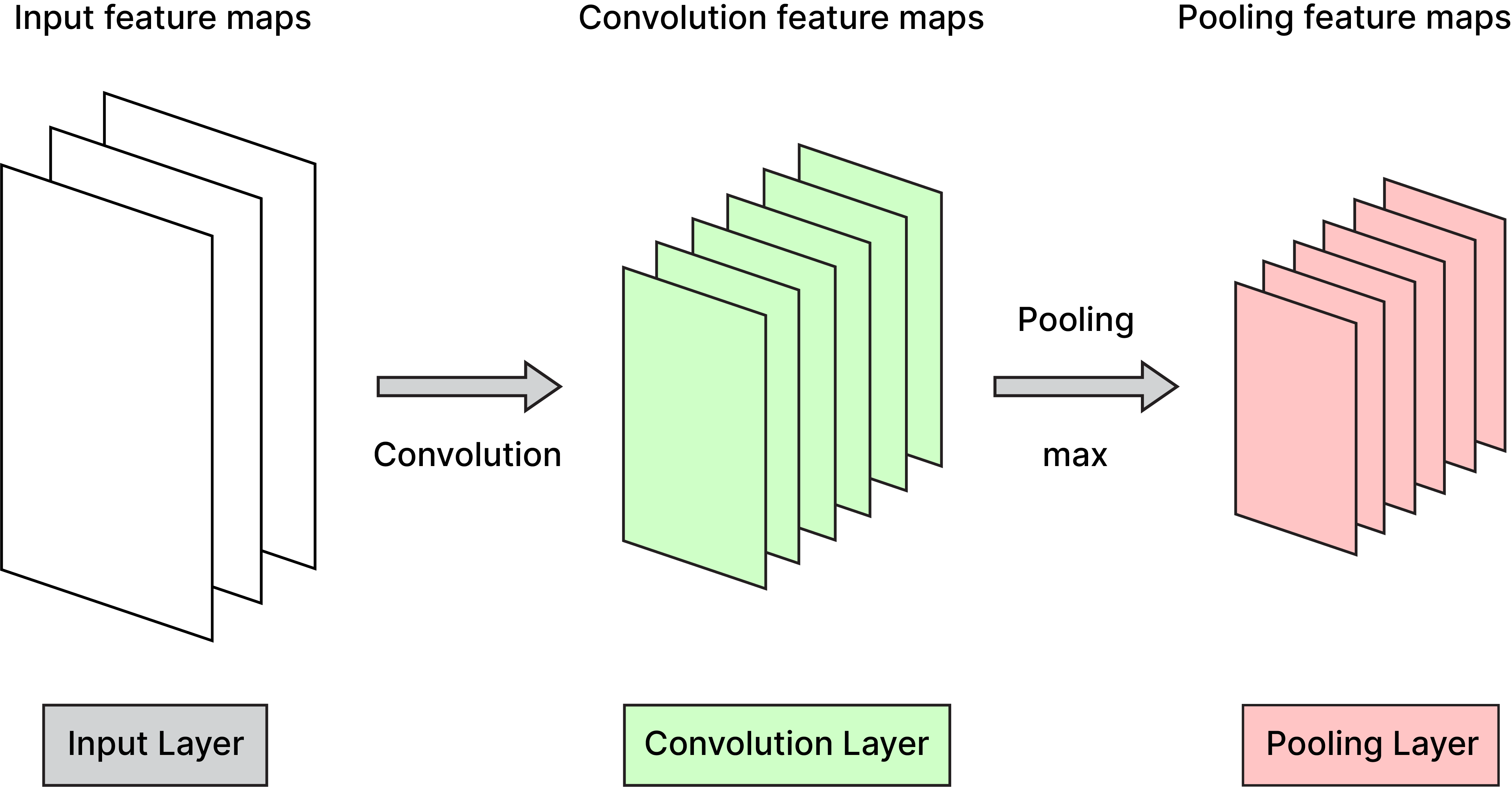## Types of pooling

There are four types of pooling procedures:

• max pooling
• average pooling
• global max pooling
• Global average pooling.
• Other rare variants like Min Pooling

All of them are for 1D, 2D or 3D layer.

#### Max pooling:

A pooling procedure known as "max pooling" chooses the largest element possible from the feature map area that the filter has covered. As a result, the output of the max-pooling layer would be a feature map that contained the most noticeable features from the prior feature map. When added to a model max-pooling reduces the dimensionality of images by reducing the number of pixels in the output from the previous convolutional layer. We can see an example: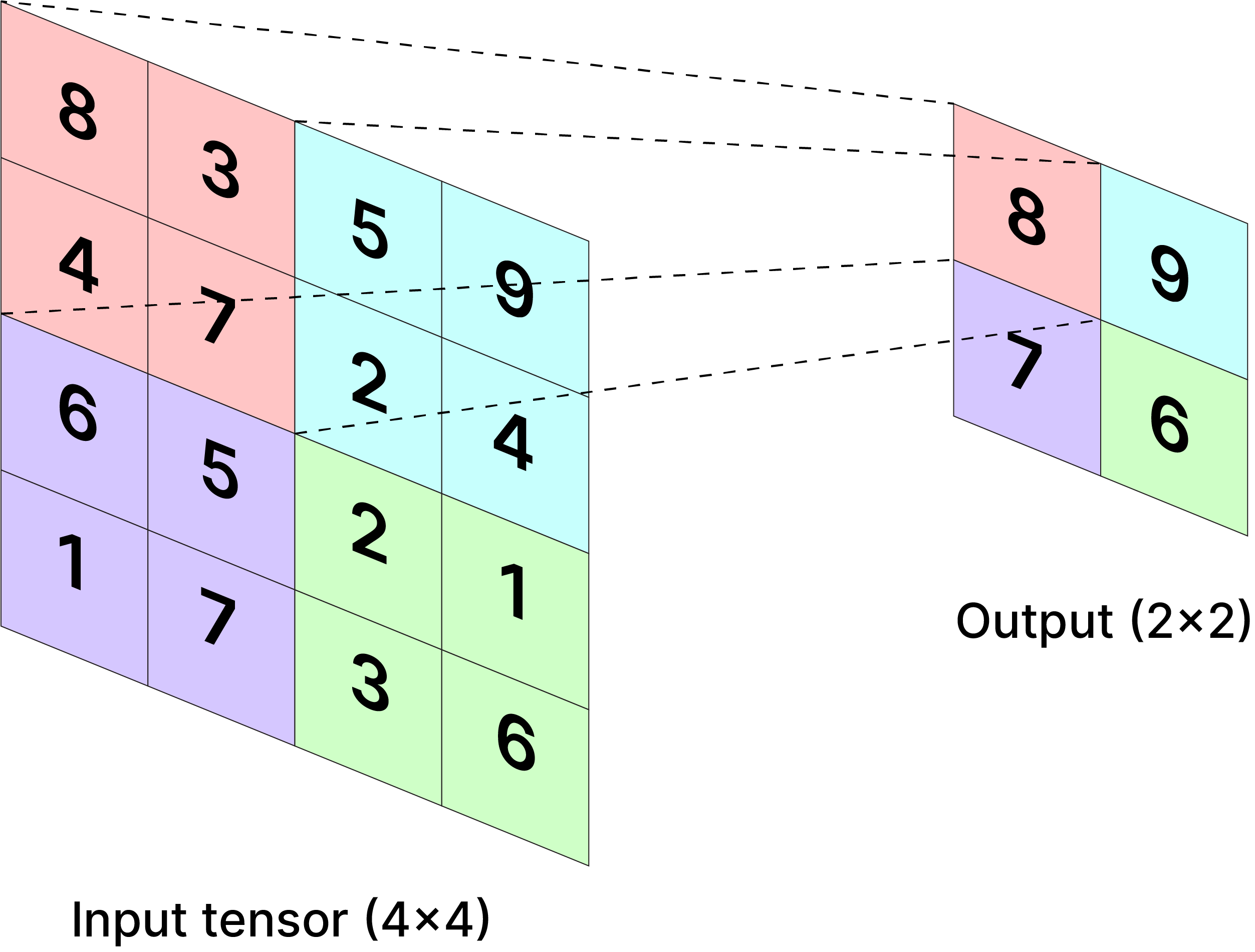#### Average pooling:

One of the types of pooling that isn’t used very often is average pooling, instead of taking the max within each filter we take the average. It does the same task as max pooling which is to reduce the dimensionality of images. We can see an example: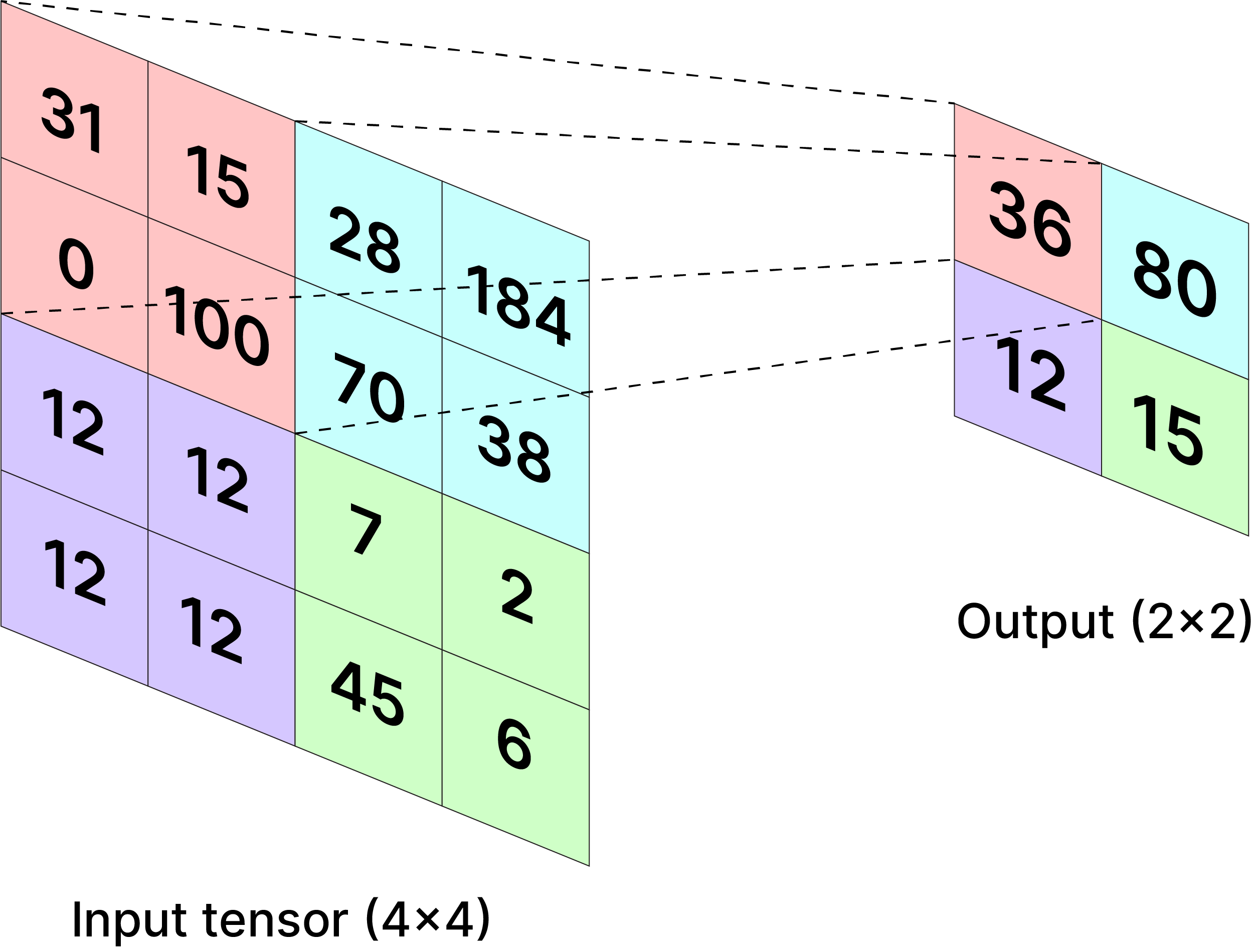#### Global Average pooling:

Global Average Pooling (GAP) is a technique commonly used in convolutional neural networks (CNNs) for dimensionality reduction in the spatial dimensions of feature maps. It is typically applied the convolutional and activation layers in a CNN architecture.

#### Global Max Pooling:

Global Max Pooling (GMP) is another technique commonly used in convolutional neural networks (CNNs) for dimensionality reduction in the spatial dimensions of feature maps. It is similar to Global Average Pooling (GAP), but instead of taking the average, it takes the maximum value of each feature map across its entire spatial extent.

## Global Average Pooling

Global Pooling condenses all of the feature maps into a single one, pooling all of the relevant information into a single map that can be easily understood by a single dense classification layer instead of multiple layers. It's typically applied as average pooling (GlobalAveragePooling2D) or max pooling (GlobalMaxPooling2D) and can work for 1D and 3D input as well.

Global Average Pooling is a pooling operation designed to replace flatten layer and fully connected layers in classical CNNs. The idea is to generate one feature map for each corresponding category of the classification task in the last layer. Usually the feature maps of the last convolutional layer are vectorized and fed into fully connected layers followed by a softmax logistic regression layer. This structure bridges the convolutional structure with traditional neural networks. Global average pooling replaces the strategy. It takes the average of each feature map and feeds the resulting vector directly into the softmax layer rather than constructing fully connected layers on top of the feature maps.

Here is the related pseudocode:

1. Take an numpy array image and define its values
2. Loop for each value of image
3. Add each element to a variable which will store sum
4. After loop finishes, divide sum with number of array elements in the feature map
5. Store that result and display

The size of feature matrix is MxN and we get only one value. Thus, the size of resultant matrix is 1x1.

The code can be done like this:

``````import numpy as np
from keras.models import Sequential
from keras.layers import GlobalAveragePooling2D

# define input image
image = np.array([[2., 2, 7, 3],
[9, 4, 6, 1],
[8, 5, 2, 4],
[3, 1, 2, 6]])
image = image.reshape(1, 4, 4, 1)

# define ga_model containing just a single global-average pooling layer
ga_model = Sequential(
[GlobalAveragePooling2D()])

# generate pooled output
ga_output = ga_model.predict(image)

# print output image
ga_output = np.squeeze(ga_output)
print("ga_output: ", ga_output)
``````

The output will be the average of the image array which is 4.0625.

## Flatten layer vs global average pooling

Most CNN architectures comprise of three segment:

• Convolutional Layers
• Pooling Layers
• Fully-Connected Layers
Fully-connected layers refer to a flattening layer and (usually) multiple dense layers.

Flattening is used to convert all the resultant arrays of any shape from pooled feature maps into a single long continuous linear vector keeping all values. It is used to make the multidimensional input one-dimensional, commonly used in the transition from the convolution layer to the full connected layer. For example a tensor (samples, 8, 10, 64) will be flattened to (samples, 8 * 10 * 64). Like this: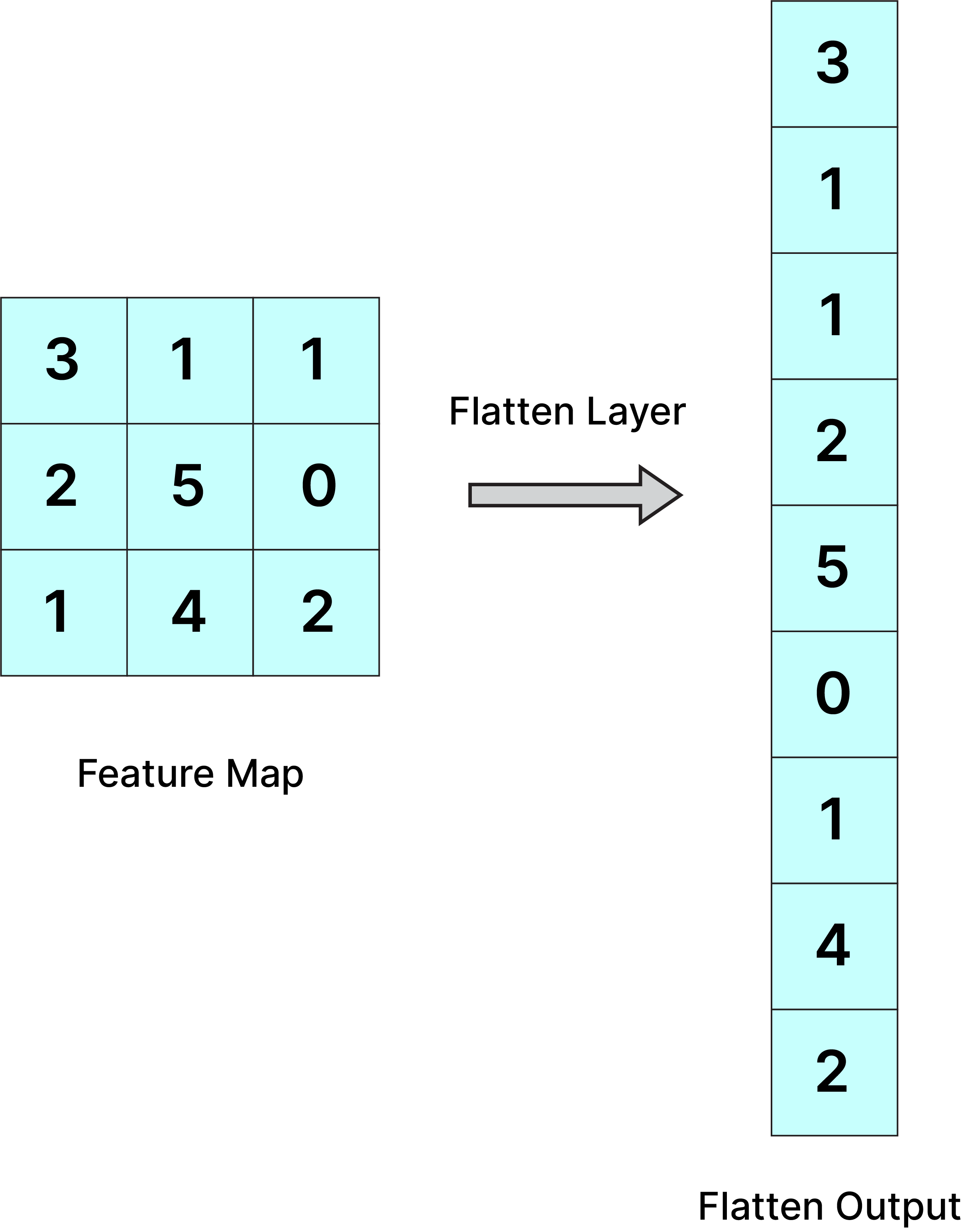Global Average Pooling does something different. It applies average pooling on the spatial dimensions until each spatial dimension is one, and leaves other dimensions unchanged. For example, a tensor (samples, 8, 10, 64) would be output as (samples, 1, 1, 64). Example: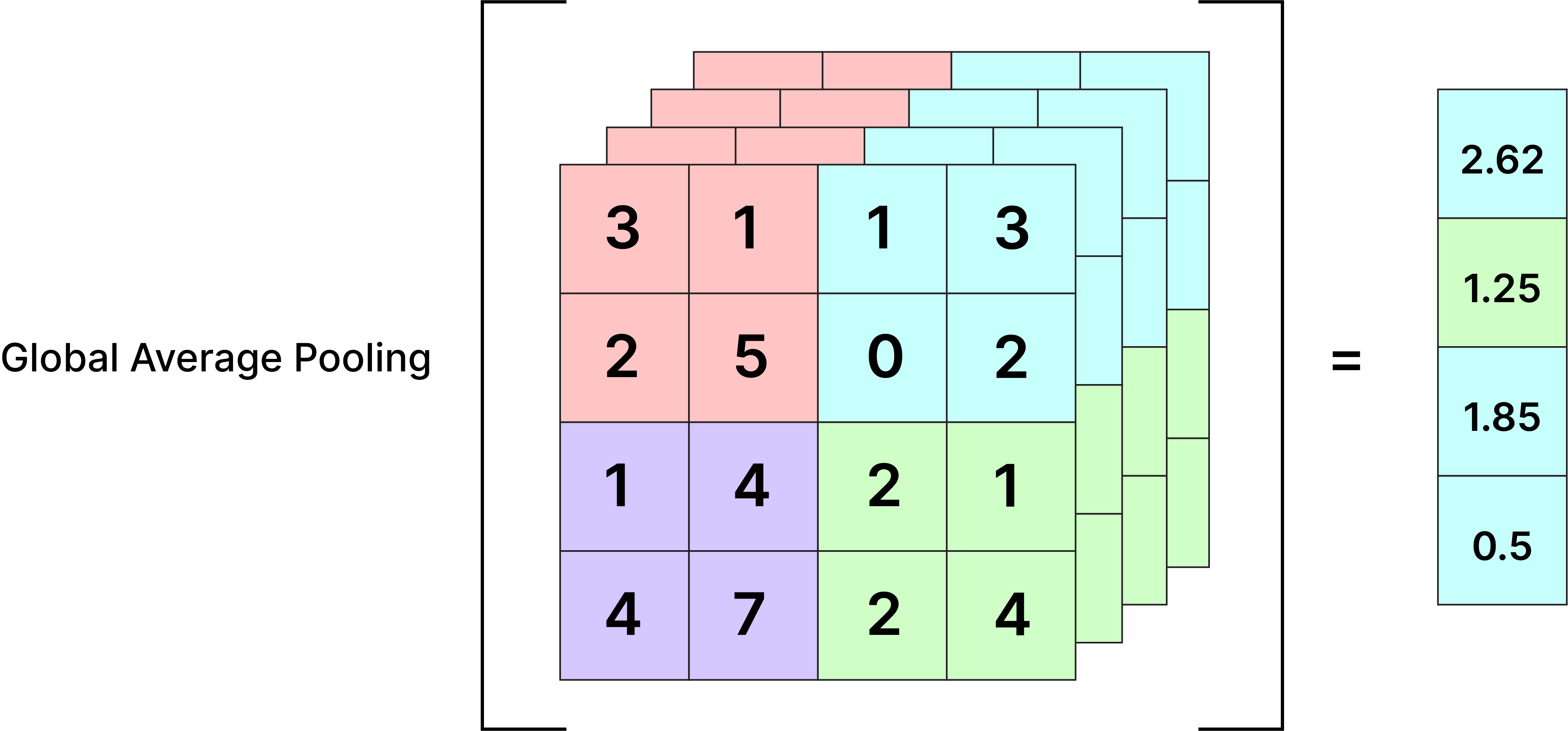## Average pooling vs global average pooling

Average pooling layer gives the largest value in a certain subarea as an output, while the global average pooling does this in the whole area.
Average pooling downsamples patches of the input feature map to reduce computation. Whereas, global pooling downsamples the entire feature map to a single value.
The average pooling function in keras uses three hyperparameters which are: filter size, stride and padding. Global average pooling doesn't take any hyperparameter. We can understand, Global max pooling = ordinary max pooling layer with pool size equals to the size of the input.
Global pooling can be used in a model to aggressively summarize the presence of a feature in an image. It is also sometimes used in models as an alternative to using a fully connected layer to transition from feature maps to an output prediction for the model. But, ordinary pooling is used in a model only to downsample or summarize the presence of a feature in an image.
For example, if the input of the max pooling layer is 1,1,2,2,5,1,6, global max pooling outputs 5, whereas ordinary max pooling layer with pool size equals to 3 outputs 2,2,5,5,6assuming stride=1).

## Advantages of global average pooling

1. One advantage of GAP is that there is no parameter to optimize in the global average pooling thus overfitting is avoided at this layer.
2. Global average pooling sums out the spatial information, thus it is more robust to spatial translations of the input.

## Comparing different pooling function with code

``````import numpy as np
from keras.models import Sequential
from keras.layers import AveragePooling2D
from keras.layers import GlobalMaxPooling2D
from keras.layers import GlobalAveragePooling2D, MaxPooling2D

# define input image
image = np.array([[2., 2, 7, 3],
[9, 4, 6, 1],
[8, 5, 2, 4],
[3, 1, 2, 6]])
image = image.reshape(1, 4, 4, 1)

# define model containing just a single average pooling layer
model = Sequential(
[AveragePooling2D(pool_size = 2, strides = 2)])

# generate pooled output
output = model.predict(image)

# print output image
output = np.squeeze(output)
print("Avergae pooling:\n", output)
print(output.shape)

model = Sequential(
[MaxPooling2D(pool_size = 2, strides = 2)])

# generate pooled output
output = model.predict(image)

# print output image
output = np.squeeze(output)
print("Max pooling:\n", output)
print(output.shape)

gm_model = Sequential(
[GlobalMaxPooling2D()])

# define ga_model containing just a single global-average pooling layer
ga_model = Sequential(
[GlobalAveragePooling2D()])

# generate pooled output
gm_output = gm_model.predict(image)
ga_output = ga_model.predict(image)

# print output image
gm_output = np.squeeze(gm_output)
ga_output = np.squeeze(ga_output)
print("Global max pooling output: ", gm_output)
print("Global average pooling output: ", ga_output)
print(ga_output.shape)
``````

The output is:
Avergae pooling:
[[4.25 4.25]
[4.25 3.5 ]]
Shape:
(2, 2)
Max pooling:
[[9. 7.]
[8. 6.]]
Shape:
(2, 2)
Global max pooling output: 9.0
Global average pooling output: 4.0625